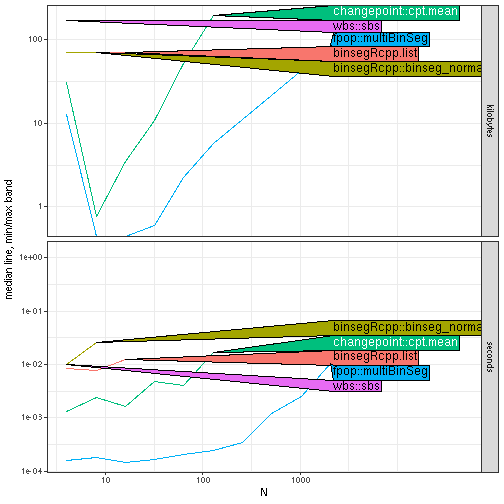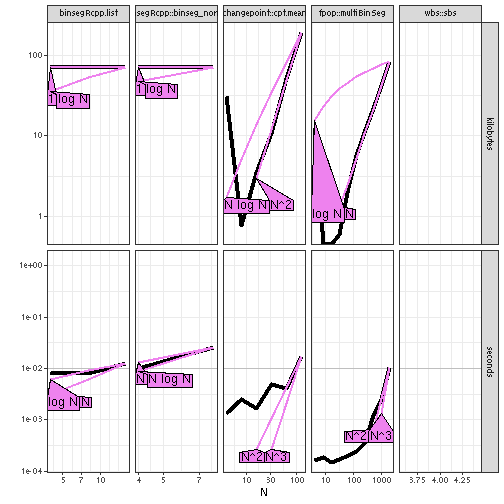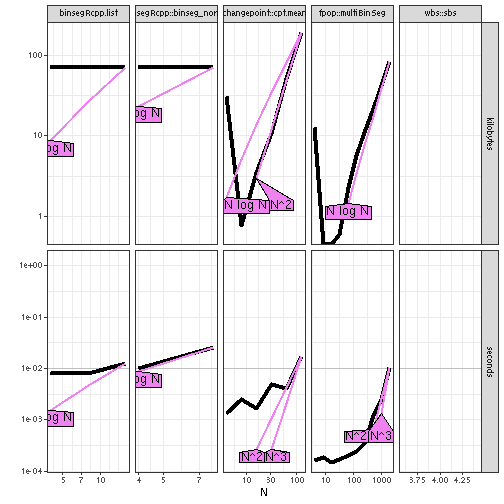# Custom References

The goal of this vignette is to show how to use custom asymptotic references. As an example, we explore the differences in asymptotic time complexity between different implementations of binary segmentation.

## Asymptotic time/memory measurement of different R expressions

The code below uses the following arguments:

• `N` is a numeric vector of data sizes,
• `setup` is an R expression to create the data,
• the other arguments have names to identify them in the results, and values which are R expressions to time,
``````library(data.table)
seg.result <- atime::atime(
N=2^seq(2, 20),
setup={
max.segs <- as.integer(N/2)
max.changes <- max.segs-1L
set.seed(1)
data.vec <- 1:N
},
"changepoint::cpt.mean"={
cpt.fit <- changepoint::cpt.mean(data.vec, method="BinSeg", Q=max.changes)
sort(c(N,cpt.fit@cpts.full[max.changes,]))
},
"binsegRcpp::binseg_normal"={
binseg.fit <- binsegRcpp::binseg_normal(data.vec, max.segs)
sort(binseg.fit\$splits\$end)
},
"fpop::multiBinSeg"={
mbs.fit <- fpop::multiBinSeg(data.vec, max.changes)
sort(c(mbs.fit\$t.est, N))
},
"wbs::sbs"={
wbs.fit <- wbs::sbs(data.vec)
split.dt <- data.table(wbs.fit\$res)[order(-min.th, scale)]
sort(split.dt[, c(N, cpt)][1:max.segs])
},
binsegRcpp.list={
binseg.fit <- binsegRcpp::binseg(
"mean_norm", data.vec, max.segs, container.str="list")
sort(binseg.fit\$splits\$end)
})
plot(seg.result)
#> Warning: Transformation introduced infinite values in continuous y-axis
#> Transformation introduced infinite values in continuous y-axis
#> Transformation introduced infinite values in continuous y-axis
#> Warning in grid.Call.graphics(C_polygon, x\$x, x\$y, index): semi-transparency is
#> not supported on this device: reported only once per page
``````The plot method creates a log-log plot of median time and memory vs data size, for each of the specified R expressions. The plot above shows some speed differences between binary segmentation algorithms, but they could be even easier to see for larger data sizes (exercise for the reader: try modifying the `N` and `seconds.limit` arguments). You can also see that memory usage is much larger for changepoint than for the other packages.

You can use `references_best` to get a tall/long data table that can be plotted to show both empirical time and memory complexity:

``````seg.best <- atime::references_best(seg.result)
plot(seg.best)
#> Warning: Transformation introduced infinite values in continuous y-axis
#> `geom_line()`: Each group consists of only one observation.
#> ℹ Do you need to adjust the group aesthetic?
#> `geom_line()`: Each group consists of only one observation.
#> ℹ Do you need to adjust the group aesthetic?
#> Warning in grid.Call.graphics(C_polygon, x\$x, x\$y, index): semi-transparency is
#> not supported on this device: reported only once per page
``````The figure above shows asymptotic references which are best fit for each expression. We do the best fit by adjusting each reference to the largest N, and then ranking each reference by distance to the measurement of the second to largest N.

## Custom asymptotic references

If you have one or more expected time complexity classes that you want to compare with your empirical measurements, you can use the `fun.list` argument. Note that each function in that list should take as input the data size `N` and output log base 10 of the reference function, as below:

``````my.refs <- list(
"N \\log N"=function(N)log10(N) + log10(log(N)),
"N^2"=function(N)2*log10(N),
"N^3"=function(N)3*log10(N))
my.best <- atime::references_best(seg.result, fun.list=my.refs)
plot(my.best)
#> Warning: Transformation introduced infinite values in continuous y-axis
#> `geom_line()`: Each group consists of only one observation.
#> ℹ Do you need to adjust the group aesthetic?
#> `geom_line()`: Each group consists of only one observation.
#> ℹ Do you need to adjust the group aesthetic?
#> Warning in grid.Call.graphics(C_polygon, x\$x, x\$y, index): semi-transparency is
#> not supported on this device: reported only once per page
``````From the plot above you should be able to see the asymptotic time complexity class of each algorithm. Keep in mind that the plot method displays only the next largest and next smallest reference lines, from those specified in `fun.list`.

• the fastest packages are log-linear (fpop, wbs, binsegRcpp),
• that the binsegRcpp implementation that uses the list container is much slower (quadratic).
• the changepoint package uses a super-quadratic algorithm (actually cubic, which you could see if you increase N even larger).

• increase `seconds.limit` to see the differences more clearly.